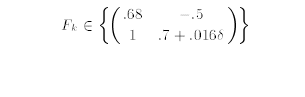# Robust kalman filter

hi i’m new in cvx ,and i want to solve this problem. i have a robust kalman filter with uncertainty in F
x(k+1)=Fx(k)+Gu
y(k+1)=Hx(k)+V
.The
uncertain state matrices F are assumed to lie inside the convex polytope as F=[and the vertices of polytope are F1=[0.65 -0.5 ; 1 0.7016] and F2=[0.65 -0.5 1 0.684]
how can i explain F as code?